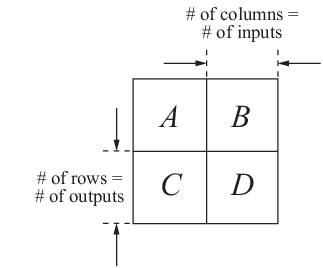## MIMO State-Space Models

### MIMO Explicit State-Space Models

You create a MIMO state-space model in the same way as you create a SISO state-space model. The only difference between the SISO and MIMO cases is the dimensions of the state-space matrices. The dimensions of the B, C, and D matrices increase with the numbers of inputs and outputs as shown in the following illustration.In this example, you create a state-space model for a rotating body with inertia tensor J, damping force F, and three axes of rotation, related as:

`$\begin{array}{c}J\frac{d\omega }{dt}+F\omega =T\\ y=\omega .\end{array}$`

The system input T is the driving torque. The output y is the vector of angular velocities of the rotating body.

To express this system in state-space form:

`$\begin{array}{c}\frac{dx}{dt}=Ax+Bu\\ y=Cx+Du\end{array}$`

rewrite it as:

`$\begin{array}{c}\frac{d\omega }{dt}=-{J}^{-1}F\omega +{J}^{-1}T\\ y=\omega .\end{array}$`

Then the state-space matrices are:

`$A=-{J}^{-1}F,\text{ }B={J}^{-1},\text{ }C=I,\text{ }D=0.$`

To create this model, enter the following commands:

```J = [8 -3 -3; -3 8 -3; -3 -3 8]; F = 0.2*eye(3); A = -J\F; B = inv(J); C = eye(3); D = 0; sys_mimo = ss(A,B,C,D);```

These commands assume that J is the inertia tensor of a cube rotating about its corner, and the damping force has magnitude 0.2.

`sys_mimo` is an `ss` model.

### MIMO Descriptor State-Space Models

This example shows how to create a continuous-time descriptor (implicit) state-space model using `dss`.

This example uses the same rotating-body system shown in MIMO Explicit State-Space Models, where you inverted the inertia matrix J to obtain the value of the B matrix. If J is poorly-conditioned for inversion, you can instead use a descriptor (implicit) state-space model. A descriptor (implicit) state-space model is of the form:

`$\begin{array}{c}E\frac{dx}{dt}=Ax+Bu\\ y=Cx+Du\end{array}$`

Create a state-space model for a rotating body with inertia tensor J, damping force F, and three axes of rotation, related as:

`$\begin{array}{c}J\frac{d\omega }{dt}+F\omega =T\\ y=\omega .\end{array}$`

The system input T is the driving torque. The output y is the vector of angular velocities of the rotating body. You can write this system as a descriptor state-space model having the following state-space matrices:

`$A=-F,\text{ }B=I,\text{ }C=I,\text{ }D=0,\text{ }E=J.$`

To create this system, enter:

```J = [8 -3 -3; -3 8 -3; -3 -3 8]; F = 0.2*eye(3); A = -F; B = eye(3); C = eye(3); D = 0; E = J; sys_mimo = dss(A,B,C,D,E) ```

These commands assume that J is the inertia tensor of a cube rotating about its corner, and the damping force has magnitude 0.2.

`sys` is an `ss` model with a nonempty E matrix.

### State-Space Model of Jet Transport Aircraft

This example shows how to build a MIMO model of a jet transport. Because the development of a physical model for a jet aircraft is lengthy, only the state-space equations are presented here. See any standard text in aviation for a more complete discussion of the physics behind aircraft flight.

The jet model during cruise flight at MACH = 0.8 and H = 40,000 ft. is

```A = [-0.0558 -0.9968 0.0802 0.0415 0.5980 -0.1150 -0.0318 0 -3.0500 0.3880 -0.4650 0 0 0.0805 1.0000 0]; B = [ 0.0073 0 -0.4750 0.0077 0.1530 0.1430 0 0]; C = [0 1 0 0 0 0 0 1]; D = [0 0 0 0]; ```

Use the following commands to specify this state-space model as an LTI object and attach names to the states, inputs, and outputs.

```states = {'beta' 'yaw' 'roll' 'phi'}; inputs = {'rudder' 'aileron'}; outputs = {'yaw rate' 'bank angle'}; sys_mimo = ss(A,B,C,D,'statename',states,... 'inputname',inputs,... 'outputname',outputs); ```

You can display the LTI model by typing `sys_mimo`.

```sys_mimo ```
``` a = beta yaw roll phi beta -0.0558 -0.9968 0.0802 0.0415 yaw 0.598 -0.115 -0.0318 0 roll -3.05 0.388 -0.465 0 phi 0 0.0805 1 0 b = rudder aileron beta 0.0073 0 yaw -0.475 0.0077 roll 0.153 0.143 phi 0 0 c = beta yaw roll phi yaw rate 0 1 0 0 bank angle 0 0 0 1 d = rudder aileron yaw rate 0 0 bank angle 0 0 Continuous-time model. ```

The model has two inputs and two outputs. The units are radians for `beta` (sideslip angle) and `phi` (bank angle) and radians/sec for `yaw` (yaw rate) and `roll` (roll rate). The rudder and aileron deflections are in degrees.

As in the SISO case, use `tf` to derive the transfer function representation.

```tf(sys_mimo) ```
``` Transfer function from input "rudder" to output... -0.475 s^3 - 0.2479 s^2 - 0.1187 s - 0.05633 yaw rate: --------------------------------------------------- s^4 + 0.6358 s^3 + 0.9389 s^2 + 0.5116 s + 0.003674 0.1148 s^2 - 0.2004 s - 1.373 bank angle: --------------------------------------------------- s^4 + 0.6358 s^3 + 0.9389 s^2 + 0.5116 s + 0.003674 Transfer function from input "aileron" to output... 0.0077 s^3 - 0.0005372 s^2 + 0.008688 s + 0.004523 yaw rate: --------------------------------------------------- s^4 + 0.6358 s^3 + 0.9389 s^2 + 0.5116 s + 0.003674 0.1436 s^2 + 0.02737 s + 0.1104 bank angle: --------------------------------------------------- s^4 + 0.6358 s^3 + 0.9389 s^2 + 0.5116 s + 0.003674 ```